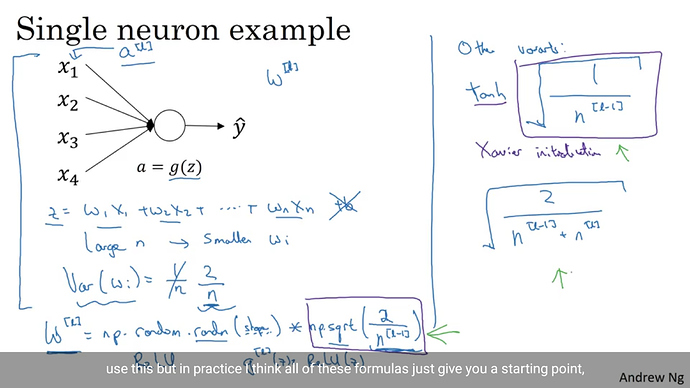# Initialise weight for each layer using random values

How to Initialise weight for each layer using random values, such as shown in this images belowWhich initialization would you want to perform?
From Andrew’s slides it looks like you sample from a normal distribution (`torch.randn`) and multiply with different scales, i.e.
`torch.sqrt(2/n_in)`, `torch.tanh(torch.sqrt(1/n_in))`, and `torch.sqrt(2/(n_in+n_out))`.

You can find the initialization methods like `xavier_normal` here.

How to fix those weights for all the layers

`torch.sqrt(2/n_in)`

this is the more general code if i am not wrong

``````for m in self.modules():
if isinstance(m, nn.Conv2d) or isinstance(m, nn.Linear):
import scipy.stats as stats
stddev = m.stddev if hasattr(m, 'stddev') else 0.1
X = stats.truncnorm(-2, 2, scale=stddev)
values = torch.Tensor(X.rvs(m.weight.data.numel()))
values = values.view(m.weight.data.size())
m.weight.data.copy_(values)
elif isinstance(m, nn.BatchNorm2d):
m.weight.data.fill_(1)
m.bias.data.zero_()
``````

How do I find number of inputs for each layer?

You can use _calculate_fan_in_and_fan_out from `init.py` to get the number of input and output units.

``````import torch.nn.init as init
#inside the main class where the Convolution, Linear Layers and BatchNorm are defined
for m in self.modules():
if isinstance(m,nn.Conv2d) or isinstance(m,nn.Linear):
in_v,_ = init._calculate_fan_in_and_fan_out() #what should be tensor that goes in here
X = torch.randn(m.weight.data.size())*torch.sqrt(2/in_v)
values = torch.Tensor(X.rvs(m.weight.data.numel()))
values = values.view(m.weight.data.size())
m.weight.data.copy_(values)
elif isinstance(m,nn.BatchNorm2d):
m.weight.data.fill_(1)
m.bias.data.zero_()
``````

What should be feed inside the `init._calculate_fan_in_and_fan_out()`?
Is this code correct?

You should pass your parameter into this function, e.g.:

``````in_v, out_v = init._calculate_fan_in_and_fan_out(m.weight)
``````

Besides that the code won’t work as it seems you are trying to call a `scipy.stats` function on `X`.
`X.rvs` is not defined, since `X` will be a tensor sampled from the normal distribution.